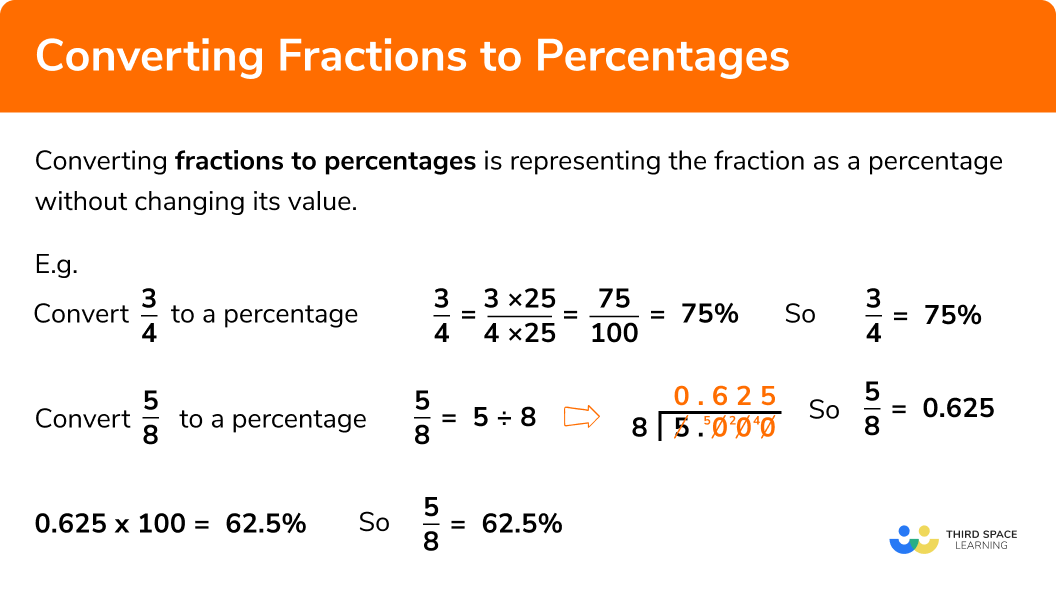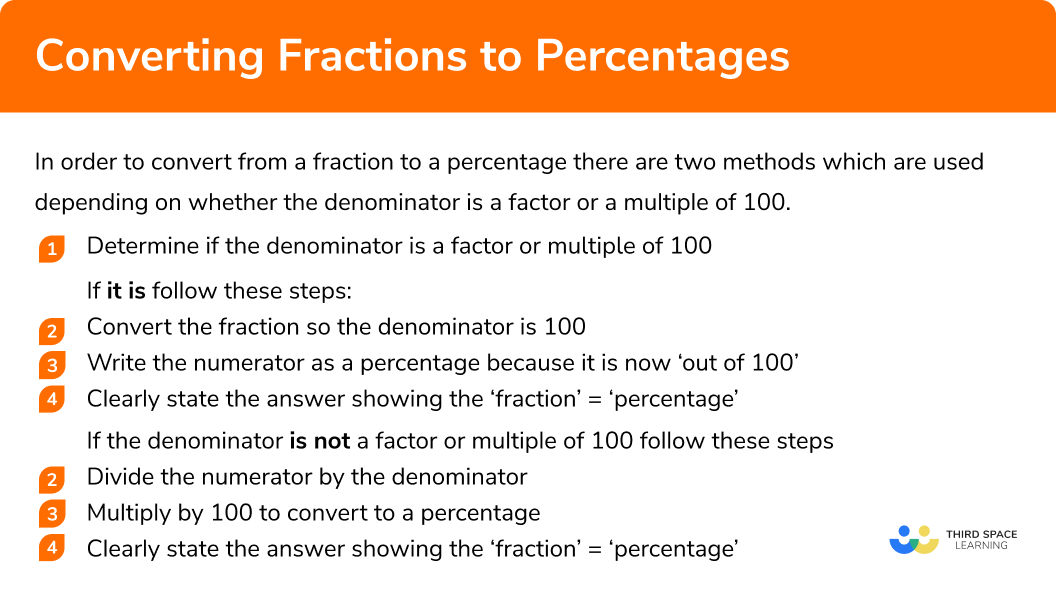GCSE Maths Number FDP Converting FDP

Fractions To Percentages

# Fractions To Percentages

Here we will learn about converting fractions to percentages.

There are also fractions to percentages worksheets based on Edexcel, AQA and OCR exam questions, along with further guidance on where to go next if you’re still stuck.

## What is converting fractions to percentages?

Converting fractions to percentages is representing the fraction as a percentage without changing its value.

E.g.

\begin{aligned} \frac{1}{4}&=25 \% \\\\ \frac{9}{20}&=45 \% \\\\ \frac{1}{3}&=33.\dot{3} \% \\\\ \frac{4}{5}&=80 \% \end{aligned}

### What is converting fractions to percentages?## How to convert fractions to percentages

In order to convert from a fraction to a percentage there are two methods which are used depending on whether the denominator is a factor or a multiple of 100.

1. Determine if the denominator is a factor or multiple of 100

If it is follow these steps:

2Convert the fraction so the denominator is 100

3Write the numerator as a percentage because it is now ‘out of 100

4Clearly state the answer showing the ‘fraction’ = ‘percentage’

If the denominator is not a factor or multiple of 100 follow these steps:

2Divide the numerator by the denominator

3Multiply by 100 to convert to a percentage

4Clearly state the answer showing the ‘fraction’ = ‘percentage’

### Explain how to convert fractions to percentages## Converting fractions to percentages examples

### Example 1: converting a simple fraction to a percentage, where the denominator is a factor of 100

Convert \frac{3}{4} to a percentage

1. See if the denominator is a factor or multiple of 100

4 is a factor of 100 (because 4 × 25 =100)

2 Convert the fraction so the denominator is 100

4 needs to be multiplied by 25 to make 100 so we are going to multiply the denominator and numerator by 25 (this ensures they are equivalent fractions).

\begin{aligned} &\frac{3}{4} \\\\ &=\frac{3\times25}{4\times25} \\\\ &=\frac{75}{100} \\\\ \end{aligned}

3Write the numerator as a percentage

4Clearly state the answer showing the ‘fraction’ = ‘percentage’

$\frac{3}{4}=75\%$

### Example 2: converting a fraction to a percentage, where the denominator is a multiple of 100

Convert \frac{60}{200} to a percentage

200 is a multiple of 100 (because 200 ÷ 2  = 100)

200 needs to be divided by 2 to make 100 so we are going to divide the denominator and numerator by 2

\begin{aligned} &\frac{60}{200}\\\\ &=\frac{60\div2}{200\div2}\\\\ &=\frac{30}{100} \end{aligned}

Because we are converting to a percentage we only need to simplify the fraction so that the denominator is 100. The fraction is not in its simplest form.

$\frac{60}{200}=30\%$

### Example 3: converting a fraction to a percentage, where the denominator is not a factor/multiple of 100

Convert \frac{5}{8} to a percentage

8 is not a factor or multiple of 100

\begin{aligned} &\frac{5}{8} = 5\div8 \\\\ \end{aligned}

Therefore,

$\frac{5}{8}=0.625$

\begin{aligned} &0.625\times100 \\\\ &62.5\% \end{aligned}
$\frac{5}{8}=62.5\%$

### Example 4: converting a fraction to a percentage, where the denominator is not a factor/multiple of 100 – involving a recurring decimal

Convert \frac{2}{9} to a percentage

9 is not a factor or multiple of 100

\begin{aligned} &\frac{2}{9}=2\div9\\\\ \end{aligned}

Therefore,

$\frac{2}{9}=0.22222…=0.\dot{2}$
$0.\dot{2}\times100 = 22.\dot{2}\%$

$\frac{2}{9}=22.\dot{2}\%$

### Example 5: converting an improper fraction to a percentage

Convert \frac{25}{20} to a percentage

20 is a multiple of 100 (because 20 × 5 =100)

20 needs to be multiplied by 5 to make 100 so we are going to multiply the denominator and numerator by 5

\begin{aligned} &\frac{25}{20}\\\\ &=\frac{25\times5}{20\times5}\\\\ &=\frac{125}{100} \end{aligned}

$\frac{25}{20}=125\%$

### Example 6: converting a mixed number to a percentage

Convert 4\frac{5}{16} to a percentage

Added step- convert to an improper fraction first:

$4\frac{5}{16}=\frac{69}{16}$

16 is not a factor or multiple of 100

\begin{aligned} &\frac{69}{16} = 69\div16 \\\\ \end{aligned}

Therefore

$\frac{69}{16}=4.3125$
\begin{aligned} &4.3125\times100 \\\\ &431.25\% \end{aligned}
$\frac{69}{16}=431.25\%$

### Calculator skills

If you are allowed to use a calculator you can perform the operation in one calculation.

E.g.
Convert \frac{25}{20} to a percentage

1. Enter the fraction on the calculator and multiply by 100
2. Press =
3. This means 125\%

### Common misconceptions

• Mistakes with written division

Often mistakes are made when implementing a form of written division. For example a common mistake with the ‘bus stop’ method is mixing up the number being divided (dividend) by the number you are dividing by (divisor).

The numerator is the dividend and therefore goes inside the ‘bus stop’.

• Not multiplying by 100 to make a percentage

The percent sign means the number is given out of 100 therefore we need to multiply the result of the division (the quotient) by 100 when writing it as a percentage.

E.g

$\frac{3}{4}=0.75=75\%$

• Not noticing a recurring decimal

Sometimes a recurring decimal is not immediately obvious. For example

$\frac{1}{7}=0.142857142857142857..$

Therefore

$\frac{1}{7}= 0.\dot{1}4285\dot{7}$

Fractions to percentages is part of our series of lessons to support revision on comparing fractions, decimals and percentages. You may find it helpful to start with the main comparing fractions, decimals and percentages lesson for a summary of what to expect, or use the step by step guides below for further detail on individual topics. Other lessons in this series include:

### Practice converting fractions to percentages questions

1. Convert \frac{1}{10} to a percentage

1\%10\%0.1\%0.01\%\begin{aligned} 1\div10=0.1\\\\ 0.1\times100=10\\\\ 10\%\\ \end{aligned}

2. Convert \frac{4}{10} to a percentage

40\%4\%0.4\%2.5\%\begin{aligned} 4\div10=0.4\\\\ 0.4\times100=40\\\\ 40\% \end{aligned}

3. Convert \frac{11}{10} to a percentage

90.9\%1.1\%\frac{11}{10}\%110\%\begin{aligned} 110\div10=1.1\\\\ 1.1\times100=110\\\\ 110\% \end{aligned}

4. Convert \frac{6}{1000} to a percentage

0.6166.6\%6\%0.6\%\begin{aligned} 6\div1000=0.006\\\\ 0.006\times100=0.6\\\\ 0.6\% \end{aligned}

5. Convert \frac{601}{20} to a percentage

30.053005\%3.327787\%601\%\begin{aligned} 601\div20=30.05\\\\ 30.05\times100=3005\\\\ 3005\% \end{aligned}

6. Convert \frac{15}{16} to a percentage

0.9375\%93.75\%1.06\%937.5\%\begin{aligned} 15\div16=0.9375\\\\ 0.9375\times100=93.75\\\\ 93.75\% \end{aligned}

### Converting fractions to percentages GCSE questions

1.  Convert each of the following fractions to percentages

a) \frac{9}{10}

b) \frac{1}{4}

c) \frac{9}{100}

d) \frac{9}{1000}

e) \frac{99}{10}

(5 Marks)

a) 90\%

(1)

b) 25\%

(1)

c) 9\%

(1)

d) 0.9\%

(1)

e) 990\%

(1)

2.  Convert each of the following fractions to percentages

a) \frac{16}{50}

b) \frac{7}{25}

c) \frac{4}{75}

(4 Marks)

a) 32\%

(1)

b) 28\%

(1)

c) 1 mark for correct method but not showing the recurring decimals e.g 5.3\%

(1)

5.33333…\% or 5.\dot{3}\%

(1)

3. Match the fractions to the percentage

(5 Marks)

\frac{2}{5}=40\%

(1)

\frac{3}{4}=75\%

(1)

\frac{6}{5}=120\%

(1)

\frac{26}{10}=260\%

(1)

\frac{3}{100}=3\%

(1)

4.  Represent \frac{113}{125} as a percentage.

(2 Marks)

113\div125=0.904

(1)

0.904\times100=90.4

90.4\%

(1)

## Learning checklist

You have now learned how to:

• Convert a fraction to a percentage

## Still stuck?

Prepare your KS4 students for maths GCSEs success with Third Space Learning. Weekly online one to one GCSE maths revision lessons delivered by expert maths tutors.

Find out more about our GCSE maths tuition programme.